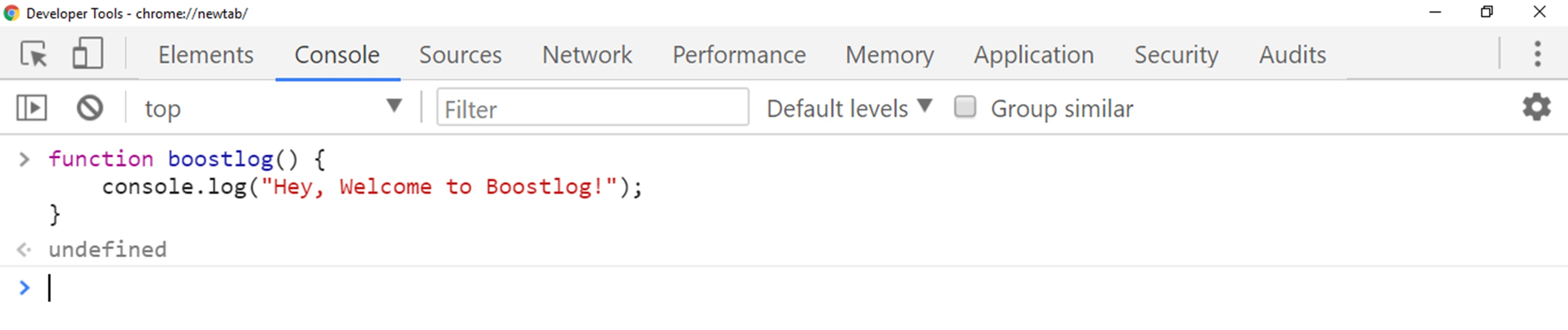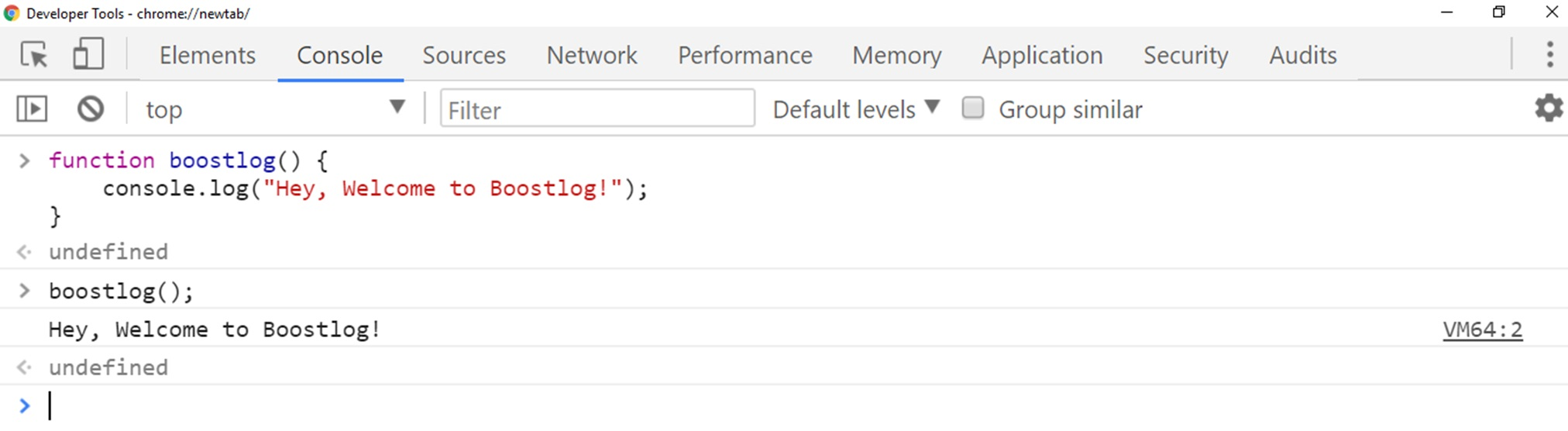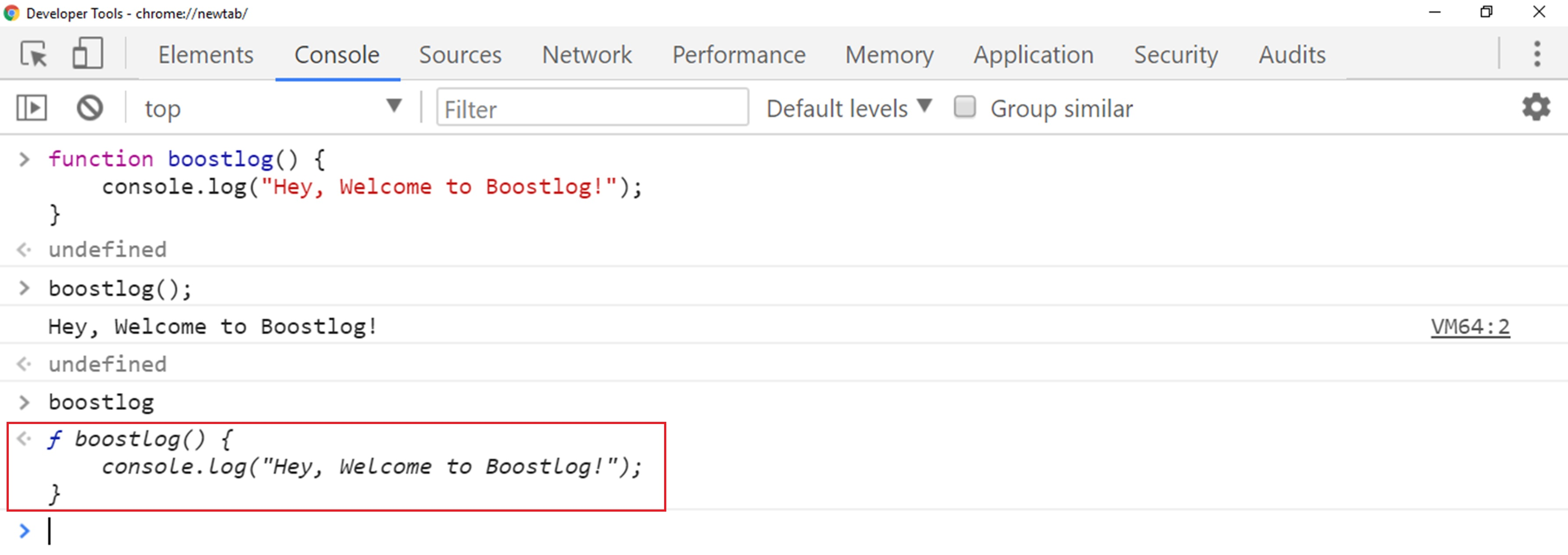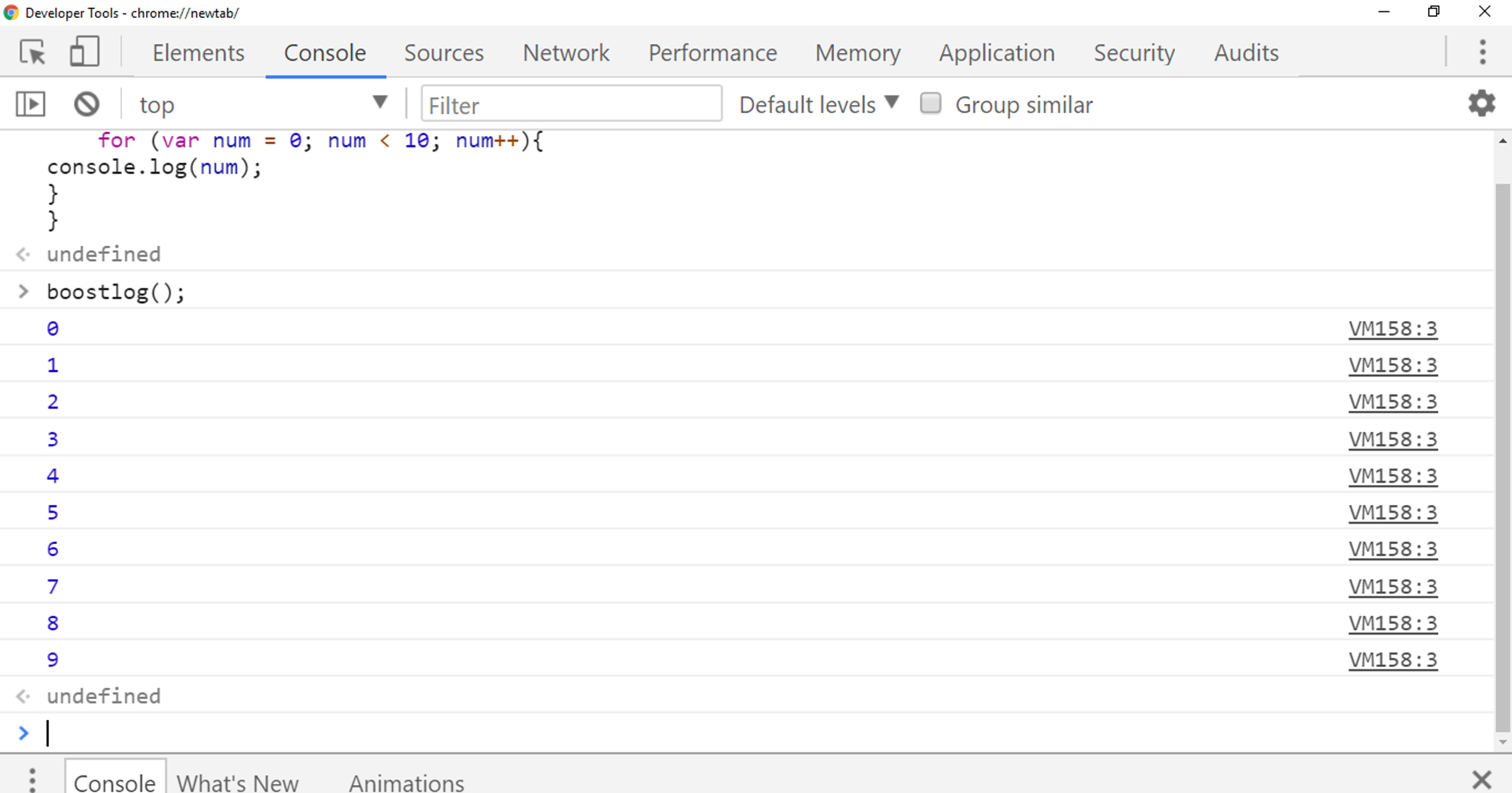# Lesson 13 Functions in JavaScript

This lesson is all about JavaScript function, and function are building blocks of JavaScript.
So in this lesson, we are going to learn another important component of JavaScript and we will discuss why we use functions and we will learn how we can declare the function.

### JavaScript Functions

Functions are one of the most important parts of JavaScript and we are beginning this lesson by talking about what functions actually are.
So functions are something like a bunch of reusable codes that perform some action or return some value.
Reusability of codes makes our program more efficient.
The function is a two-step process, we declare function first and then we call it later.
We use ‘function’ keyword to declare the function.

So it is a two-step process: Declare a function.
Then call it.

### Syntax for function in JavaScript:

So function statement declared by function keyword, followed by function name, followed by parentheses and then curly braces. Here is the syntax to declare a function in JavaScript below:

``````function ourFunctionName() {
// Bunch of code to be executed
}
``````

Here ‘function’ is a keyword just like var, while or if and ‘ourFunctionName’ is the name of our function which we use to recall function latter and it function name written as camel case, it’s something like the first word is in lower case and the second word is uppercase.

Parentheses could be empty or we can declare multiple parameters separated by commas within the parameter.
Within curly braces {} we will write the bunch of code that defines the purpose of our function.
We call the function later by referencing its name with parenthesis at the end.

### Example

So let me show you with some example that how function looks like.
So I will define a function here.

``````function boostlog() {
console.log("Hey, Welcome to Boostlog!");
}
``````

So when I declared a function with the name ‘boostlog’ in our Google Chrome JavaScript console then nothing happened, it is because we have just declared a function not recall it yet.So now if we want to call that function, we refer to its name ‘boostlog’ followed by parentheses.
Just like that:

``````boostlog();
``````

Now hit enter and you will get:So it is a simple example of the function which consists of the single line of code, so this function can be reused as many time as we want.
Note: if we write our function name ‘boostlog’ without parentheses in our Javascript console then it will not run our function statement but return back our function code.You can see in above screenshot, when we wrote function name without parentheses then it returned our function code back.
So there is a difference in referring function and executing the function.

### Example

So here is another example which will tell us how functions can help DRY (Don’t Repeat Yourself) up our code.

``````function boostlog() {
for (var num = 0; num < 10; num++){
console.log(num);
}
}
``````

So here we have declared another function with the for statement, the purpose of this code is to print number from 0 to 9 and here we have used console.log(num); to print numbers.You can notice in above output, when we called ‘boostlog’ function and it printed numbers from 0 to 9, it is because our function having for statement which runs every time until it gets the false statement and our function consists of console.log statements, which print number every time code execute.

### Conclusion

In this lesson, we have discussed why we use functions and learned how we can declare the function.
So the function is all about the bunch of reusable code that performs some task, which makes our program efficient.